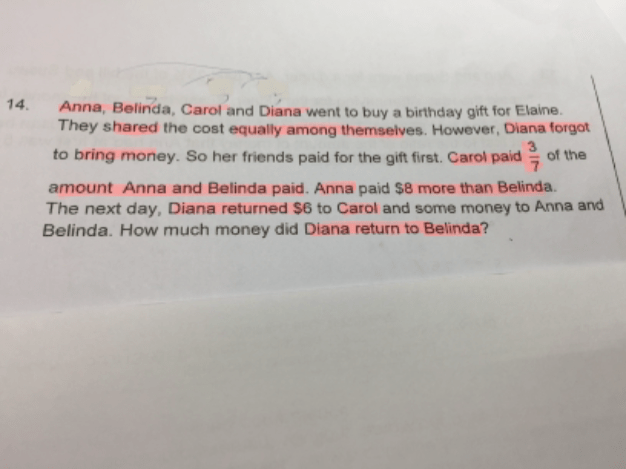# QuestionHi there, just to confirm, is the answer for this question \$3?

No, it should be \$8. My solution is as follows :

Carol : Anna + Belinda : Total
3 : 7 : 10

10/4 = 2.5 (Each share in units)
3 – 2.5 = 0.5 ——-> 6 (Diana returned \$6 to Carol)
(6/0.5) x 10 = 120 (total cost of gift)

(120/10) x 7 = 84
or
120/4 = 30
30 + 6 = 36
120 – 36 = 84

(84 – 8)/2 = 38
38 – 30 = 8

Ans : \$8.

0 Replies 0 Likes ✔Accepted Answer